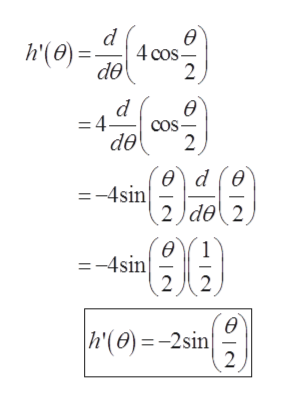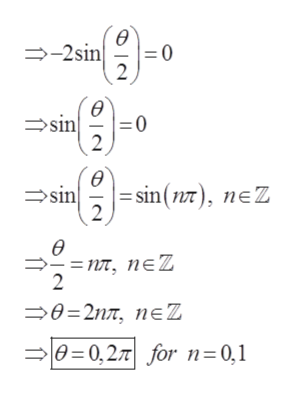# Show that the function has local extreme values at the given values of 0 and say which kind of local extrema the function has.24.h(0) 4 cos0s0s 2T, 0 = 0 and 0 2xFind h'(0)h'(0)Set h'(0) equal to 0 and solve for 0 on 0s0s 2.Ө-(Type an exact answer, using t as needed. Use a comma to separate answers as needed.)Өhas local extreme values at 02on 0 s0 s 2n.Thus, h(0) 4 cos(Type an exact answer, using t as needed. Use a comma to separate answers as needed.)Evaluate h(0) at 0 and 2t.h(0)(Simplify your answer, including any radicals. Use integers or fractions for any numbers in the expression.)h(21)(Simplify your answer, including any radicals. Use integers or fractions for any numbers in the expression.)Determine which kind of local extrema the function has at 0 0 and 0 27tat 0 2TThe function has a local (1)at 0 0 and a local (2)|(1)(2)minimummaximummaximumminimum

Question
1 views

Can you help with this problem step by step?

check_circle

Step 1

Given,

Step 2

The derivative of the function ishelp_outlineImage Transcriptioncloseө d 4 cos h'(0) de 2 ө =4- cOS cos- de 2 ө) d (0 =-4sin 2 de 2 ө =-4sin 22 ө h'(0)= -2sin 2 fullscreen
Step 3

Take the derivative is...help_outlineImage Transcriptioncloseө -2sin = 0 2 ө sin 2 ө | sin () nZ sin 2 ө пт, пеZ 2 —0-2пт, пеZ 0 0,27 for n=0,1 fullscreen

### Want to see the full answer?

See Solution

#### Want to see this answer and more?

Solutions are written by subject experts who are available 24/7. Questions are typically answered within 1 hour.*

See Solution
*Response times may vary by subject and question.
Tagged in

### Functions